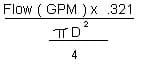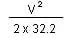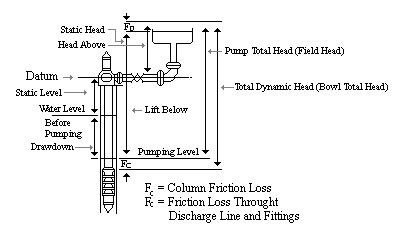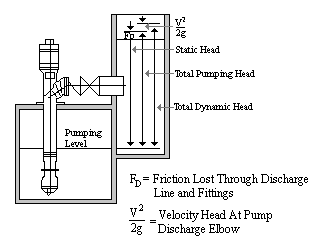Vertical Pump Nomenclature
Vertical Pump Nomenclature

BOWL EFFICIENCY (or laboratory efficiency) is the efficiency as indicated on the bowl performance curve, including any applicable corrections.

BOWL HORSEPOWER (or laboratory horsepower) is the horsepower required at the bowl shaft to deliver the required capacity against the bowl total head and is defined by the following formula:

 Bowl Bowl Total Head (feet) X Capacity (GPM) Specific Horsepower = 3960 x Bowl Efficiency x Gravity

Bowl Total Head (or laboratory head) is the head, in feet, on the pump bowl and is equal to the pump total head plus the column friction loss.

 = Pump Total Head + Column Friction Loss + Discharge Elbow

BRAKE HORSEPOWER (or field horsepower) is the horsepower required at the top shaft and is equal to the bowl horsepower plus shaft loss.

BRAKE HORSEPOWER = Bowl Horsepower + Shaft Loss

Brake Horsepower, is the horsepower required at the top of the pump to produce the desired head and capacity.

 Brake Total Head x Flow (GPM) Horsepower = 3960 x Pump Efficiency

CAPACITY is the rate of flow, in gallons per minute.

COLUMN FRICTION LOSS is the friction loss, in feet of head, through the column and is dependent upon both capacity and the length and diameter of column and shaft used. Column friction loss is indicated in feet of heat per hundred feet of column and shaft.

DATUM is the horizontal plane defined by the elevation of the bottom of the discharge head. Sometimes the centerline of discharge is referred to as the Datum and is acceptable but the vertical distance between the center line of discharge and the bottom of the discharge head should be accounted for.

DRAWDOWN is the difference, in feet, between the static water level and the pumping water level.

DRIVER EFFICIENCY is the ratio of driver output to driver input with no external thrust load, and therefore, must be adjusted to reflect thrust bearing loss.

 Driver Driver Output (nameplate rating) Efficiency = Driver Input + Thrust Bearing Loss

HEAD ABOVE DATUM is the static discharge head plus the friction loss through the discharge line and fittings plus the velocity head.

INPUT HORSEPOWER is the total power required to operate the pump and motor.

 Input Brake Horsepower Horsepower = Driver Efficiency

LIFT (HEAD) BELOW DATUM is the vertical distance, in feet, between the datum and the pumping water level.

OVERALL EFFICIENCY (wire to water efficiency) is the efficiency of the pump and motor complete.

 OVERALL EFFICIENCY = PUMP FIELD EFFICIENCY x DRIVER EFFICIENCY

PUMP EFFICIENCY is read directly from the performance curves and as the term implies is a measure of the operating efficiency of the pump.

 Total Head x Flow (GPM) Efficiency = 3960 x Brake Horsepower

PUMP FIELD EFFICIENCY is the efficiency of the complete pump with all losses accounted for.

 Pump Pump Total Head (ft.) x Field = Capacity (GPM) x Efficiency ____Specific Gravity_____ 3960 x Brake Horsepower

PUMP HEAD equals the static lift (Hs1) below the centerline of the discharge pipe, plus the required head (Hr) above the discharge. The head above the discharge must include any friction losses (H1) through the discharge piping and fittings.

Static Head = Hs1 + Hr

PUMP TOTAL HEAD is equal to the lift below datum plus head above datum. This is the head for which the customer is responsible and is exclusive of any column friction loss.

PUMPING WATER LEVEL is the vertical distance, in feet, between the datum and the water level when the pump is operating.

SHAFT LOSS is the friction loss, measured in horsepower, between the shaft and its bearings.

SPECIFIC GRAVITY is a relative term which expresses the fluid's density with reference to fresh water at 62º F.

 Specific Density of Fluid Pumped (lbs/ft3) Gravity = Density of Water (lbs/ft3)

(S.G. of water is 1.0)

STATIC DISCHARGE HEAD is the vertical distance the liquid must be raised above the datum.

STATIC WATER LEVEL is the vertical distance, in feet, between the datum and the water level when the pump is not operating.

THRUST BEARING LOSS is the friction loss, in horsepower, developed by the total thrust load on the bearing. Bearing manufacturers estimate the loss in angular contact bearings to be approximately 0.0075 HP per 100 RPM per 1000 lbs. thrust load.

TOTAL HEAD (Total Dynamic Head) is the net change in energy of the fluid being pumped through the pump. This change in energy is expressed in terms of how high the fluid has been lifted and how fast the fluid is traveling at the point, and is the combination of items 1 and 2.

TOTAL PUMP THRUST is the load that must be carried by the thrust bearing of the pump driver, electric motor, or gear drive. This thrust is composed of the weight of the rotating parts plus the hydraulic loading on these parts.

TOTAL PUMP THRUST + TOTAL HEAD x Kt + Ka + Setting (In Feet) x Ks

VELOCITY HEAD (V2/2g) - The velocity head is dependent on the flow rate and the inside diameter of the discharge elbow. This value can be taken from the chart or calculated from the following equation:

V =HV=VISCOSITY of a liquid is a measure of the internal friction tending to resist flow. The performance of vertical turbine pumps is affected when handling viscous liquids.